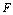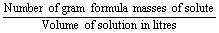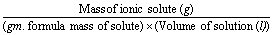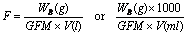1800-1023-196

+91-120-4616500

Formality

Formality (F)

Formality of a solution may be defined as the number of gram formula masses of the ionic solute dissolved per litre of the solution. It is represented by. Commonly, the term formality is used to express the concentration of the ionic solids which do not exist as molecules but exist as network of ions. A solution containing one gram formula mass of solute per litre of the solution has formality equal to one and is called formal solution. It may be mentioned here that the formality of a solution changes with change in temperature.

Formality (F)==Thus,.

NEET & AIIMS Exam Sample Papers

 AIIMS SAMPLE PAPERS View More NEET SAMPLE PAPERS View More

Get practice papers FREE

BOOK A FREE TRIAL